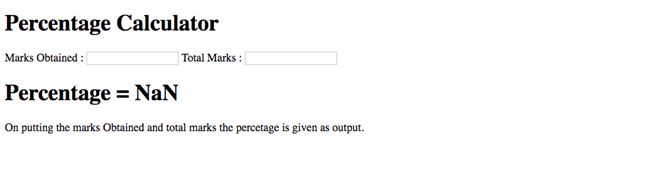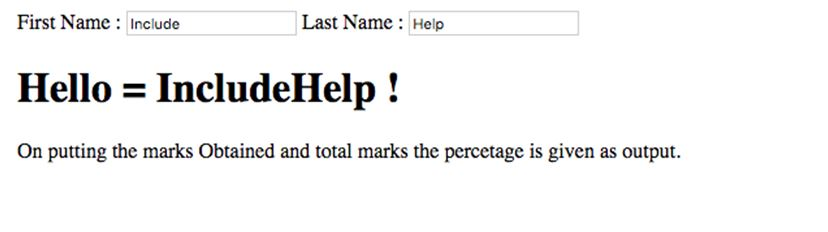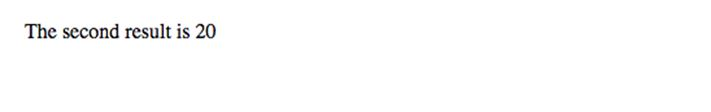# Expressions in AngularJS

AngularJS Expressions: Expression are set of operators and operands that execute to produce a value. In AngularJS an expression is calculated to give result in the calling html element. In this tutorial we will learn about expressions in AngularJS, how to use them and their example code?
Submitted by Shivang Yadav, on July 17, 2019

## AngularJS Expressions

In AngularJS, expressions are solved to give a result. It outputs the result of the expression in the html element that called it. Expressions in AngularJS contain literals, constants, operators and variables with resolve to give desired output.

Example: Angular Expression using Google CDN

```<!DOCTYPE html>
<html ng-app>

<title>Angular Js</title>
</script>

<body>
<h2>Using Angular Js : Google CDN </h2>
<hr />
<div>
{{12+15}}
</div>
</body>

</html>
```

Output

```    27
```

Code explanation:

The above code evaluates an expression in AngularJS. Using the ng-app in the html tag. This makes the whole page the owner of Angular i.e. code can be called from anywhere.

## Types of expressions in AngularJS

Expressions in AngularJS are based on the variables and literals used in the expressions,

### 1) AngularJS Number Expressions

Number can also be used in expressions. Number expressions in angular are defined and used as in the below code,

Example:

```<!DOCTYPE html>
<html>

<body>

<div ng-app="">
<h1> Percentage Calculator </h1>
<form>
Marks Obtained :
<input type="number" ng-model="marksobtained"> Total Marks :
<input type="number" ng-model="totalmarks">
</form>
<h1>Percentage = {{(marksobtained*100)/totalmarks}}</h1>
</div>

<p>On putting the marks Obtained and total marks the percetage is given as output.</p>

</body>

</html>
```

OutputThe above code takes input using form's input tag as angular variable and the does the calculations. Then prints the percentage to the <h1> tag.

### 2) AngularJS String Expressions

An expression in AngularJS can concatenate two strings to get an output string. String expressions in angular are defined and used as in the below code,

Example:

```<!DOCTYPE html>
<html>

<body>

<div ng-app="">
<h1>Hello! </h1>
<form>
First Name :
<input type="text" ng-model="firstName"> Last Name :
<input type="text" ng-model="lastName">
</form>
<h1>Hello = {{firstName + lastName }} !</h1>
</div>
<p>On putting the marks Obtained and total marks the percetage is given as output.</p>
</body>

</html>
```

Output### 3) AngularJS array Expressions

An expression in AngularJS can operate on arrays also. Array expressions in angular are defined and used as in the below code,

Example:

```<!DOCTYPE html>
<html>

<body>

<div ng-app="" ng-init="array = [10, 20, 30, 40, 50] ">
<p>The third result is {{ array }}</p>
</div>
</body>

</html>
```

OutputPreparation

What's New

Top Interview Coding Problems/Challenges!